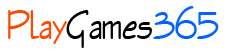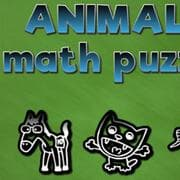enEnglishDeutschEspañolFrançaisPortuguêsРусскийItaliano# Animals Math Puzzles

## Play Animals Math Puzzles

Animals math puzzle is mathematical game with goal to solve 50 different mathematical problems all in limited time per task. Tasks are created as linear equations with 2 and 3 variables.

## How to play:

What you need to do is to find the value for the (x). (x) is a sum of the picture mark values within the (x) row. To get to (x) you need to designate a value to each picture mark according to values shown per row or column.